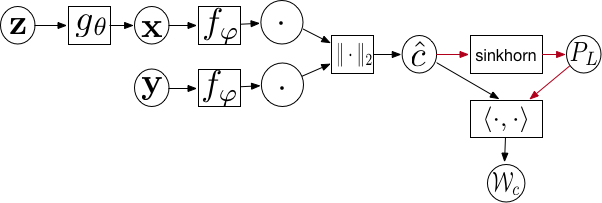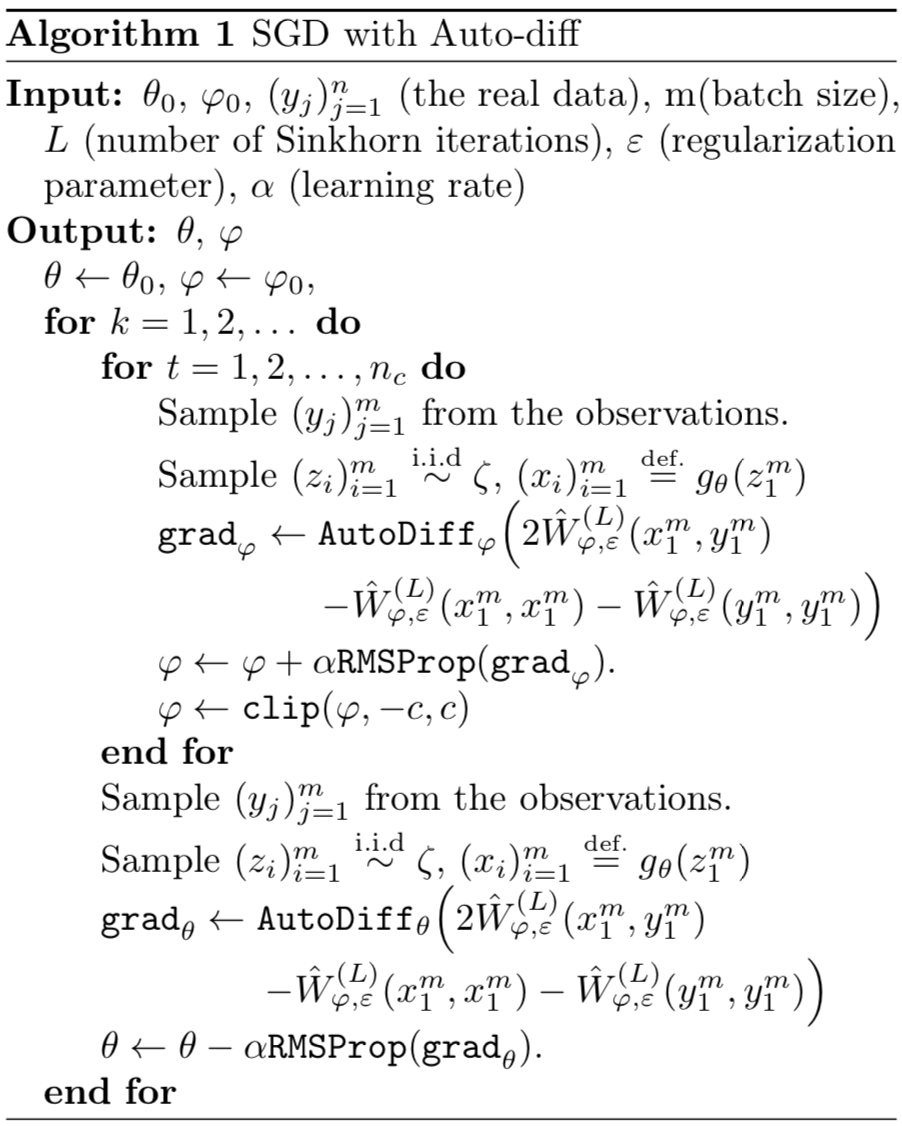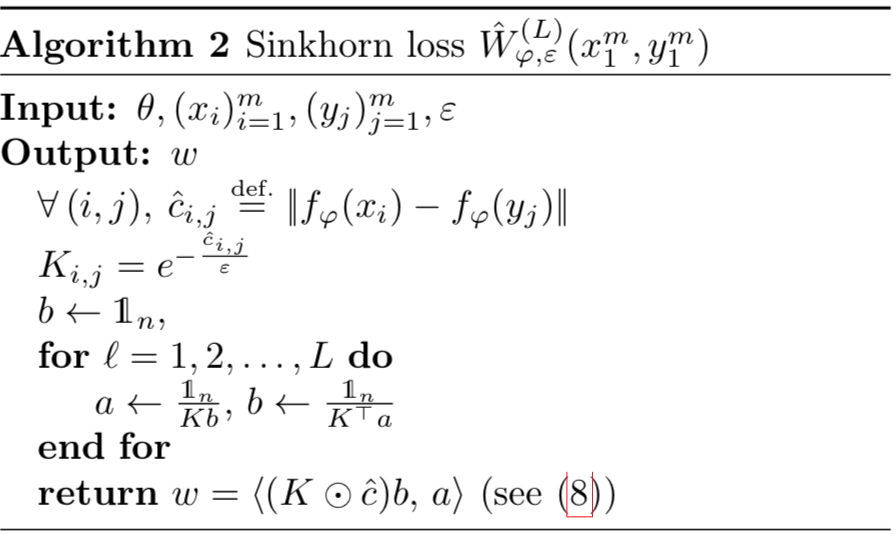Learning Generative Models with Sinkhorn Divergence@AISTATS18.

## OverviewFigure Computational Graph

From the above figure, we can see that $$P_L$$ is determined by $$\hat{c}$$; there are two flows towards $$\mathcal{W}_c$$, so we can cut off one of them while the model is still differentiable, in the sense that we can still calculate the gradients of $$\theta$$ and $$\varphi$$. Indeed, in OT-GAN, the red flow branch is cut off.

Remark: The proposed generative model actually is very similar to wgan by substituting the 1-Wasserstein loss function with Sinkhorn loss.

## Optimal transport distances

The optimal transport metric between two probability distributions $$\mu, \nu$$ supported on the metric space $$\mathcal{X}$$ is defined as the solution of the linear program:

$\mathcal{W}_c(\mu, \nu) = \min_{\pi \in \Pi(\mu, \nu)} \int_{\mathcal{X} \times \mathcal{X}} c(\mathbf{x}, \mathbf{y}) \mathrm{d} \pi(\mathbf{x}, \mathbf{y}),$

where the set of couplings is composed of joint probability distributions over the product space $$\mathcal{X} \times \mathcal{X}$$ with imposed marginals $$(\mu, \nu)$$

$\Pi(\mu, \nu) = \{ \pi: P_{1\sharp} \pi = \mu, P_{2\sharp} \pi = \nu \},$

where $$P_{1\sharp}(x, y) = x$$ and $$P_{2\sharp}(x, y) = y$$ are simple projection operators. $$c(\mathbf{x}, \mathbf{y})$$ is the ground cost to move a unit of mass form $$\mathbf{x}$$ to $$\mathbf{y}$$.

In the typical cases, the support of measure $$\mu$$ ($$\nu$$) may be a continuous manifold or very large for discrete measure, so we require to replace the initial loss function by an expectation over mini-batches of size $$(m, n)$$ and consider

\begin{align} \mu &= \frac{1}{m} \sum_{i=1}^m \delta_{\mathbf{x}_i}\\ \nu &= \frac{1}{n} \sum_{i=1}^n \delta_{\mathbf{y}_j} \end{align}

where $$\mathbf{x}_i = g_\theta (\mathbf{z}_i)$$ and $$\mathbf{y}_j$$ are instances of empirical distribution.

## Sinkhorn loss

Sinkhorn loss starts with $$b_0 = \mathbb{1}_m, \ell = 0$$, and iterates

\begin{aligned} a _ { \ell + 1 } &\stackrel { \mathrm { def. } } { = } \frac { 1 _ { n } } { K b _ { \ell } } \\ b _ { \ell + 1 } &\stackrel { \mathrm { def. } } { = } \frac { \mathbb { 1 } _ { m } } { K ^ { \top } a _ { \ell + 1 } }, \end{aligned}

where $$K_{ij} = \exp -\hat{c}_{ij}/\epsilon$$ and $$\hat{c} = [\| f_\varphi(\mathbf{x}_i) - f_\varphi(\mathbf{y}_j)\|]_{i,j} \in \mathbb{R}^{m \times n}$$. Then we have $$P_L = \operatorname { diag } \left( a _ { L } \right) K \operatorname { diag } \left( b _ { L } \right)$$, and the Sinkhorn loss $$\langle \hat{c}, P_L \rangle$$.

## Learning algorithmFigure Sinkhorn Automatic DifferentiationFigure Sinkhorn Loss